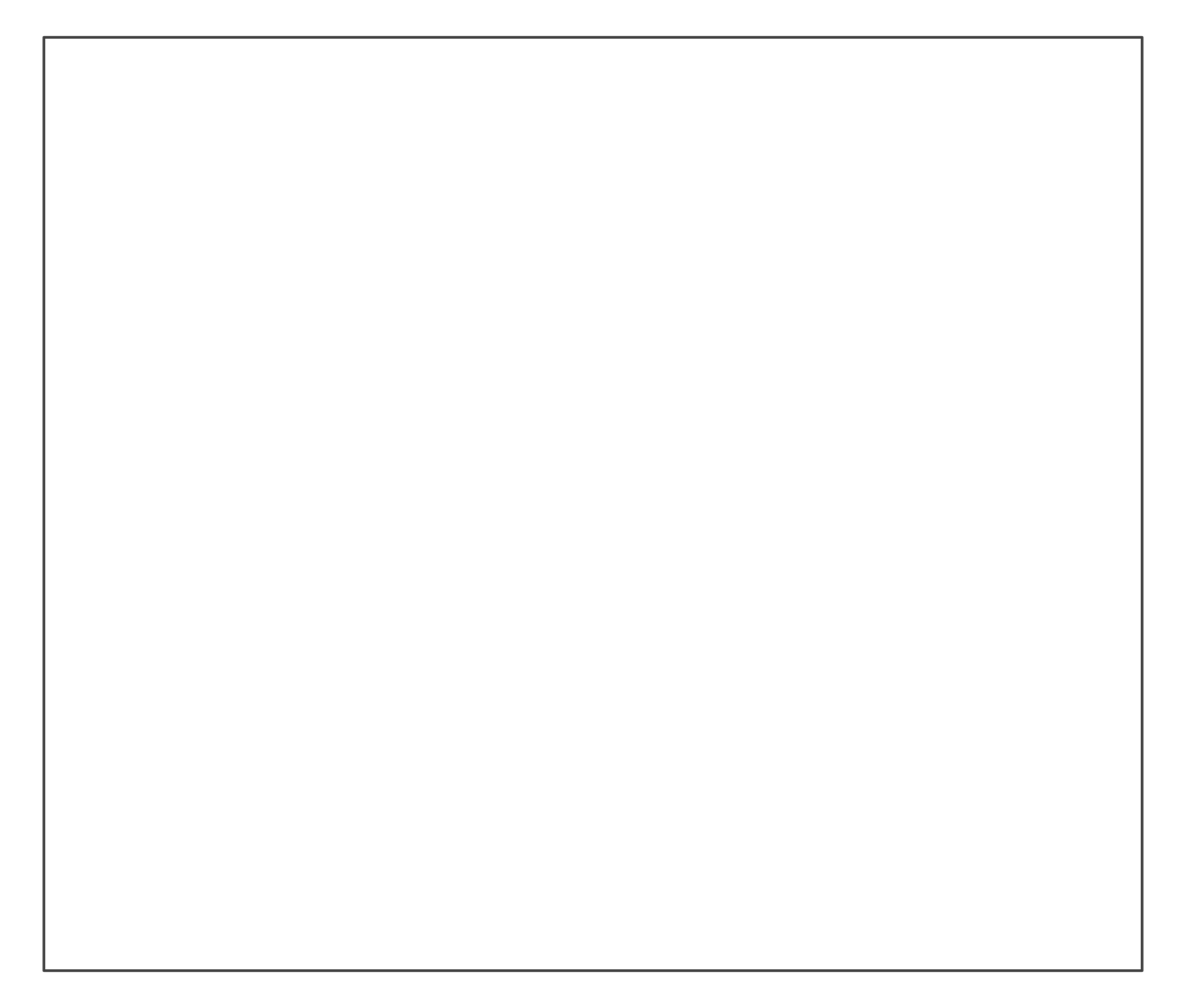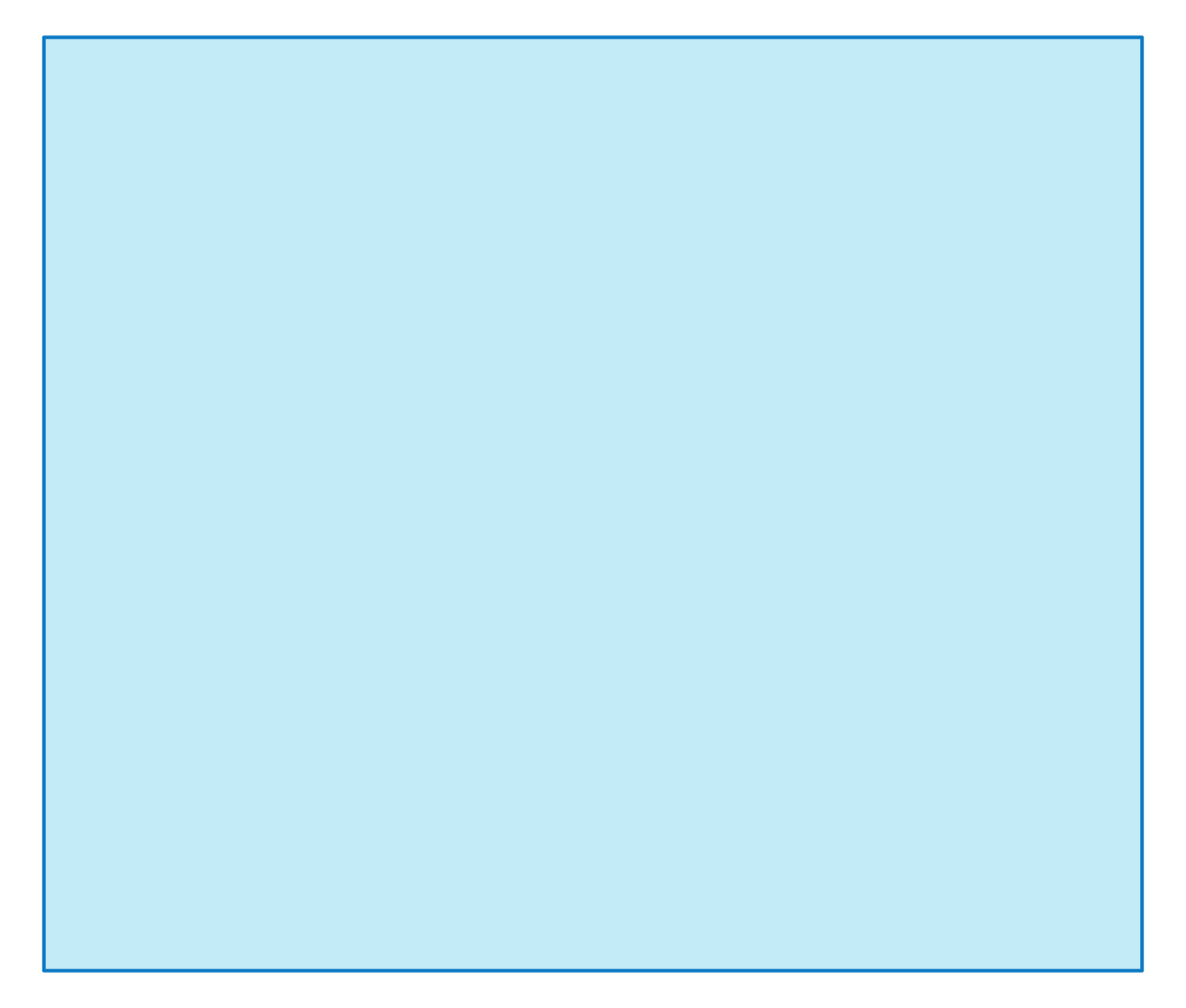slide plots an empty slide (or frame) as a colored rectangle.

slide(col = NA, dim = c(4/3, 1), border = grey(0.33, 1), lwd = 1.5)

Arguments

col The color to fill the slide with (i.e., its background color). Default: col = NA (i.e., system default for transparency). The x- and y-dimensions of the slide. Default: dim = c(4/3, 1) (i.e., unit height, 4/3 wider than high). The color of the slide's border. Setting border = NA hides border. Default: border = grey(.33, 1). The line width of the slide's border. Setting lwd = 0 or lwd = NA removes border. Default: lwd = 1.5.

heading, line, or mark to add text to a slide; xbox to plot a box.

Other plot functions: theme_grau(), theme_unikn(), xbox()

Examples

slide() # default slide (or frame)slide(lwd = NA) # borderless slide# Dimensions: slide(dim = c(18, 9)) # larger and 2:1 dimensionsslide(dim = c(1/3, 1)) # smaller and 1:3 dimensions# Formatting: slide(col = pal_seeblau[], border = pal_seeblau[], lwd = 2)